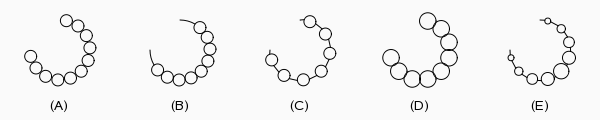# OEDrawPearlsSurfaceArc¶

bool OEDrawPearlsSurfaceArc(OEDepict::OEImageBase &image,
const OEDepict::OE2DPoint &center,
double bgnAngle, double endAngle, double radius,
const OEDepict::OEPen &pen, double edgeAngle=10.0,
double patternAngle=25.0,
double minPatternWidthRatio=0.20,
double maxPatternWidthRatio=0.20)


Draws an arc with the OESurfaceArcStyle.Pearls style.OEDrawPearlsSurfaceArc

image

The image on which the arc is drawn.

center

The center of the arc.

bgnAngle, endAngle

The two endpoints of the arc. Both angles are in degrees and their values have to be in a range of $$[0.0^{\circ} - 360.0^{\circ}]$$. Angles are interpreted such that $$0.0^{\circ}$$ and $$360.0^{\circ}$$ degrees are at the 12 o’clock position, $$90.0^{\circ}$$ degrees corresponds to 3 o’clock, etc.

The radius of the arc.

pen

The graphical properties of the arc.

edgeAngle

The angle (in degrees) between the end points of the arc and the points where the pattern of the arc starts.

See example (B) in Figure: OEDrawPearlsSurfaceArc.

patternAngle

Specifies the angle (in degrees) between the patterns of the arc. The angle has to be in a range of $$[1.0^{\circ} - 90.0^{\circ}]$$

See example (C) in Figure: OEDrawPearlsSurfaceArc.

minPatternWidthRatio, maxPatternWidthRatio

Specifies the width of the pattern of the arc relative to its radius. The min ratio is applied to the end of the arc while the max ratio specifies the width of the patterns at the middle of the arc. Both ratios have to be in a range of $$[0.01 - 1.00]$$.

See examples (D) and (E) in Figure: OEDrawPearlsSurfaceArc.